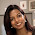### Network Theorems (Thevenin's, Superposition, Maximum Power Transfer etc...) - Topicwise GATE Questions on Network Theory (from 2003))

2003
1. A source of angular frequency 1 rad/sec has source impedance consisting of 1 Ω resistance in series with 1 H inductance. The load that will obtain the maximum power transfer is
a) 1 Ω resistance
b) 1 Ω resistance in parallel with 1 H inductance
c) 1 Ω resistance in series with 1 F capacitor
d) 1 Ω resistance in parallel with 1 F capacitor

2005
2. The maximum power that can be transferred to the load resistor RL form the voltage source in figure is

a) 1 W
b) 10 W
c) 0.25 W
d) 0.5 W

3. For the circuit shown in figure, Thevenin's voltage and Thevenin's equivalent resistance at terminals a-b is

a) 5 V and 2 Ω
b) 7.5 V and 2.5 Ω
c) 4 V and 2 Ω
d) 3 V and 2.5 Ω

2007
4. An independent voltage source in series with an impedance Zs = Rs + j Xs delivers a maximum average power to a load impedance ZL when
a) ZL = Rs + jXs
b) ZL = Rs
c) ZL = jXs
d) ZL = Rs - jXs

5. For the circuit shown in the figure, the Thevenin's voltage and resistance looking into X-Y are

a) 4/3 volts. 2 Ω
b) 4 volts. 2/3 Ω
c) 4/3 volts. 2/3 Ω
d) 4/ volts. 2 Ω

2008
6. The Thevenin’s equivalent impedance ZTH between the nodes P and Q in the following circuit is

2009
7. An AC source of RMS voltage 20 volts with internal impedance Zi = (1+2j) Ω feeds a load of impedance ZL = (7+4j) Ω in the figure below. The reactive power consumed by the load is

a) 8 VAR
b) 16 VAR
c) 28 VAR
d) 32 VAR

8. In the circuit shown, what value of RL maximizes the power delivered to RL?

a) 2.4 Ω
b) 8/3 Ω
c) 4 Ω
d) 6 Ω

2011
9. In the circuit shown below, find the value of RL such that the power transferred to RL is maximum.

a) 5 ohms
b) 10 ohms
c) 15 ohms
d) 20 ohms

2012
10. The average power delivered to an impedance (4-j3)Ω by a current 5cos(100πt+100) Amp is
a) 44.2 W
b) 50 W
c) 62.5 W
d) 125 W

11. The impedance looking into nodes 1 and 2 in the given circuit is

a) 50 Ω
b) 100 Ω
c) 5 kΩ
d) 10.1 kΩ

12. Assuming both the voltage sources are in phase, the value of R for which maximum power is transferred from circuit A to circuit B is

a) 0.8 Ω
b) 1.4 Ω
c) 2 Ω
d) 2.8 Ω

2013
13. A source Vs(t) = V cos100πt has an internal impedance of (4 + j3) Ω. If a purely resistive load connected to this source has to extract the maximum power out of the source, its value in Ω should be
a) 3
b) 4
c) 5
d) 7

14. In the circuit shown below, if the source voltage Vs = 100∟53.13o volts then the thevenin's equivalent voltage in volts as seen by the load resistance RL is

2014
15. For maximum power transfer between two cascaded sections of an electrical network, the relationship between the output impedance Z1 of the first section to the input impedance Z2 of the second section is
a) Z2 = Z1
b) Z2 = – Z1
c) Z2 = Z1*
d) Z2 = – Z1*

16. A 230 volts rms source supplies power to two loads connected in parallel. The first load draws 10kW at 0.8 leading power factor and the second one draws 10 kVA at 0.8 lagging power factor. The complex power delivered by the source is
a) (18 + j 1.5) kVA
b) (18 – j 1.5) kVA
c) (20 + j 1.5) kVA
d) (20 – j 1.5) kVA

17. Norton's theorem states that a complex network connected to a load can be replaced with an equivalence impedance
a) in series with a current source
b) in parallel with a voltage source
c) in series with a voltage source
d) in parallel with a current source

18. In the circuit shown in the figure, the angular frequency ω (in rad/sec), at which the Norton equivalent impedance as seen from terminals b – b' is purely resistive, is _____________

19. The equivalent resistance in the infinite ladder network shown in the figure is Re.

The value of Re/R is ________________
2015

20. In the given circuit, the maximum power (in Watts) that can be transferred to the load RL is _______

21. In the circuit shown, the Norton equivalent resistance (in Ω) across terminals a and b is ________

22. For the current shown in the figure, the Thevenin’s equivalent voltage (in volts) across terminals a and b is _______________

1.Thanku very much. This has been very useful.

1.2.Very usiful

3.Thank you very much sir

4.Name

Email *

Message *